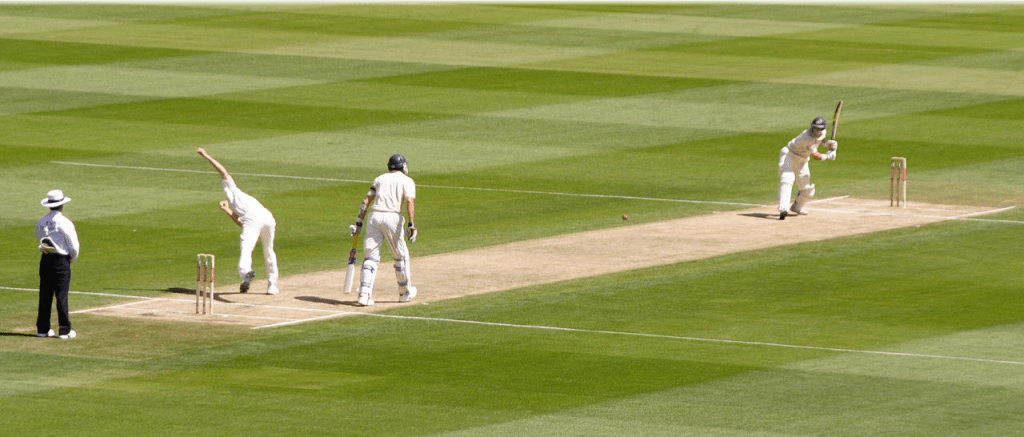# Page 3 model: The Duckworth–Lewis method

Meet the Duckworth-Lewis method, 23, from LancashireIf there are two things that typify an English summer, they are cricket and rainy days. Unfortunately, the two very often come together, which makes it very difficult to decide who should win a limited overs cricket match when rain stops play.

In these cases, a statistical model known as the Duckworth–Lewis method, devised by statistician Frank Duckworth and mathematician Tony Lewis, settles the issue (and provokes copious debate amongst Lord’s Long
Room members as they sip their champagne).The arrival of rain could reduce the teams’ batting resources—a combination of the balls left to bowl ($u$) and the number of players not out ($10 — w$). A team’s remaining resources can be modelled by the first equation on the scoreboard, where $F(w)$ is the proportion of runs you would expect to score with $w$ wickets lost compared to with no wickets lost; and $b$ is an exponential decay constant. These are both calculated from historical cricket data.
If the rain stops, the target score, $T$, can be updated using the other two formulae. Here $S$ is the number of runs obtained by Team 1; $G$ is the average score expected from the team batting first in an uninterrupted match,
published annually in the ICC Playing Handbook; and $R_1$ and $R_2$ represent the resource percentage relative to a full innings available to each team respectively, calculated using the first formula.
Now where’s that champagne?